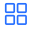Seaborn的6个简单技巧大数据频道

0

0

### 基线图

``````# 导入包
import matplotlib.pyplot as plt
import seaborn as sns

# 导入数据
df
````````````# 图
sns.scatterplot(data=df, x='body_mass_g', y='bill_length_mm',
alpha=0.7, hue='species', size='gender')
``````### 技巧

``````#

图
sns.scatterplot(data=df, x='body_mass_g', y='bill_length_mm',
alpha=0.7, hue='species', size='gender');
````````````# 图
plt.figure(figsize=(9, 5))
sns.scatterplot(data=df, x='body_mass_g', y='bill_length_mm',
alpha=0.7, hue='species', size='gender');
````````````# 图
plt.figure(figsize=(9, 5))
sns.scatterplot(data=df, x='body_mass_g', y='bill_length_mm',
alpha=0.7, hue='species', size='gender')
plt.legend(loc='upper right', bbox_to_anchor=(1.2, 1));
````````````# 更改默认样式
sns.set_style('whitegrid')

# 图
plt.figure(figsize=(9, 5))
sns.scatterplot(data=df, x='body_mass_g', y='bill_length_mm',
alpha=0.7, hue='species', size='gender')
plt.legend(loc='upper right', bbox_to_anchor=(1.2, 1));
````````````# 默认上下文更改
sns.set_context('talk')

# 图
plt.figure(figsize=(9, 5))
sns.scatterplot(data=df, x='body_mass_g', y='bill_length_mm',
alpha=0.7, hue='species', size='gender')
plt.legend(loc='upper right', bbox_to_anchor=(1.3, 1));
````````````# 更改默认调色板
sns.set_palette('rainbow')

# 图
plt.figure(figsize=(9, 5))
sns.scatterplot(data=df, x='body_mass_g', y='bill_length_mm',
alpha=0.7, hue='species', size='gender')
plt.legend(loc='upper right', bbox_to_anchor=(1.3, 1));
````````````# 更改默认调色板
sns.set_palette(['green', 'purple', 'red'])

# 图
plt.figure(figsize=(9, 5))
sns.scatterplot(data=df, x='body_mass_g', y='bill_length_mm',
alpha=0.7, hue='species', size='gender')
plt.legend(loc='upper right', bbox_to_anchor=(1.3, 1));
````````````# 更改默认调色板
sns.set_palette(['#62C370', '#FFD166', '#EF476F'])

# 图
plt.figure(figsize=(9, 5))
sns.scatterplot(data=df, x='body_mass_g', y='bill_length_mm',
alpha=0.7, hue='species', size='gender')
plt.legend(loc='upper right', bbox_to_anchor=(1.3, 1));
````````````# 导入包
import matplotlib.pyplot as plt
import seaborn as sns

# 更改默认值
sns.set_style('whitegrid')
sns.set_context('talk')
sns.set_palette('rainbow')
``````

``````# 导入包
import matplotlib.pyplot as plt
import seaborn as sns

# 更改默认值
sns.set(style='whitegrid', context='talk', palette='rainbow')
``````